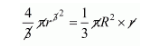# A solid sphere of radius r is melted and castQuestion:

A solid sphere of radius r is melted and cast into the shape of a solid cone of height r, the radius of the base of the cone is

(a) 2r

(b) 3r

(c) r

(d) 4r

Solution:

Volume of sphere = volume of the cone$R^{2}=4 r^{2}$

$R=2 r$

Hence, the correct answer is choice (a).